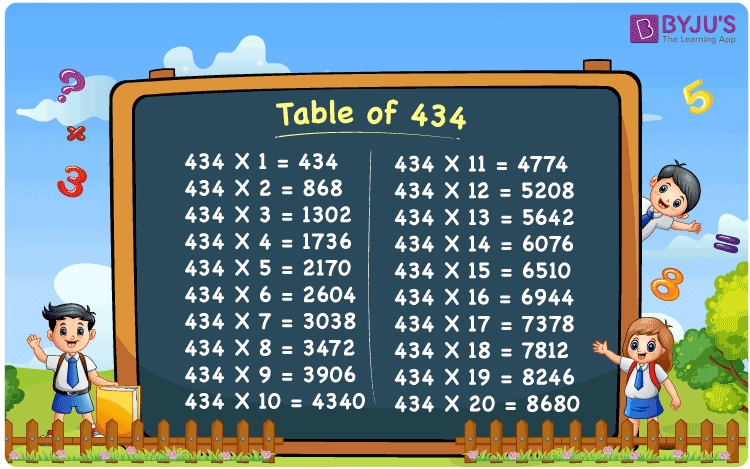Checkout JEE MAINS 2022 Question Paper Analysis : Checkout JEE MAINS 2022 Question Paper Analysis :

# Table of 434

The Table of 434 is given here up to 20 times with a solved example. Students can learn and memorise the multiplication table of 434, and quickly check the multiples of 434 while solving any problem. The table of 434 in PDF format has been also provided to download.

## Table of 434 Chart

The table of 434 chart is presented here as an attractive image chart format. This can be used as flashcards for memorising the 434 times table easily.## What is the 434 Times Table?

The 434 times table is the list of all multiples of 434 obtained by multiplying 434 with any natural number. The table of 434 can be also obtained by the repetitive addition of 434 required number of times. The below table shows the multiples of 434 in both multiplication and addition form.

 434×1 = 434 434 434×2 = 868 434 + 434 = 868 434×3 = 1302 434 + 434 + 434 = 1302 434×4 = 1736 434 + 434 + 434 + 434 = 1736 434×5 = 2170 434 + 434 + 434 + 434 + 434 = 2170 434×6 = 2604 434 + 434 + 434 + 434 + 434 + 434 = 2604 434×7 = 3038 434 + 434 + 434 + 434 + 434 + 434 + 434 = 3038 434×8 = 3472 434 + 434 + 434 + 434 + 434 + 434 + 434 + 434 = 3472 434×9 = 3906 434 + 434 + 434 + 434 + 434 + 434 + 434 + 434 + 434 = 3906 434×10 = 4340 434 + 434 + 434 + 434 + 434 + 434 + 434 + 434 + 434 + 434 = 4340

## Multiplication Table of 434

Given below is  the tabular list of multiples of 434 up to 20.

 434 × 1 = 434 434 × 2 = 868 434 × 3 = 1302 434 × 4 = 1736 434 × 5 = 2170 434 × 6 = 2604 434 × 7 = 3038 434 × 8 = 3472 434 × 9 = 3906 434 × 10 = 4340 434 × 11 = 4774 434 × 12 = 5208 434 × 13 = 5642 434 × 14 = 6076 434 × 15 = 6510 434 × 16 = 6944 434 × 17 = 7378 434 × 18 = 7812 434 × 19 = 8246 434 × 20 = 8680

## Solved Example on the Table of 434

Example 1:

How many groups of 434 items can made out of 7378 items?

Solution:

Total number of items = 7378

Number of items in each group = 434

Using the multiplication table of 434

434 × 17 = 7378

Therefore, 17 such groups can be made.

## Frequently Asked Questions on Table of 434

### What is the 434 times table?

The 434 times table is the list of all multiples of 434 starting from 434 × 1 = 434, 434 × 2 = 868, 434 × 3 = 1302, 434 × 4 = 1736, 434 × 5 = 2170 and so on.

### Which times table has 434?

434 comes in the times tables of 1, 2, 7, 14, 31, 62, 217 and 434.

### What is 7 times 434?

7 times 434 is 3038.